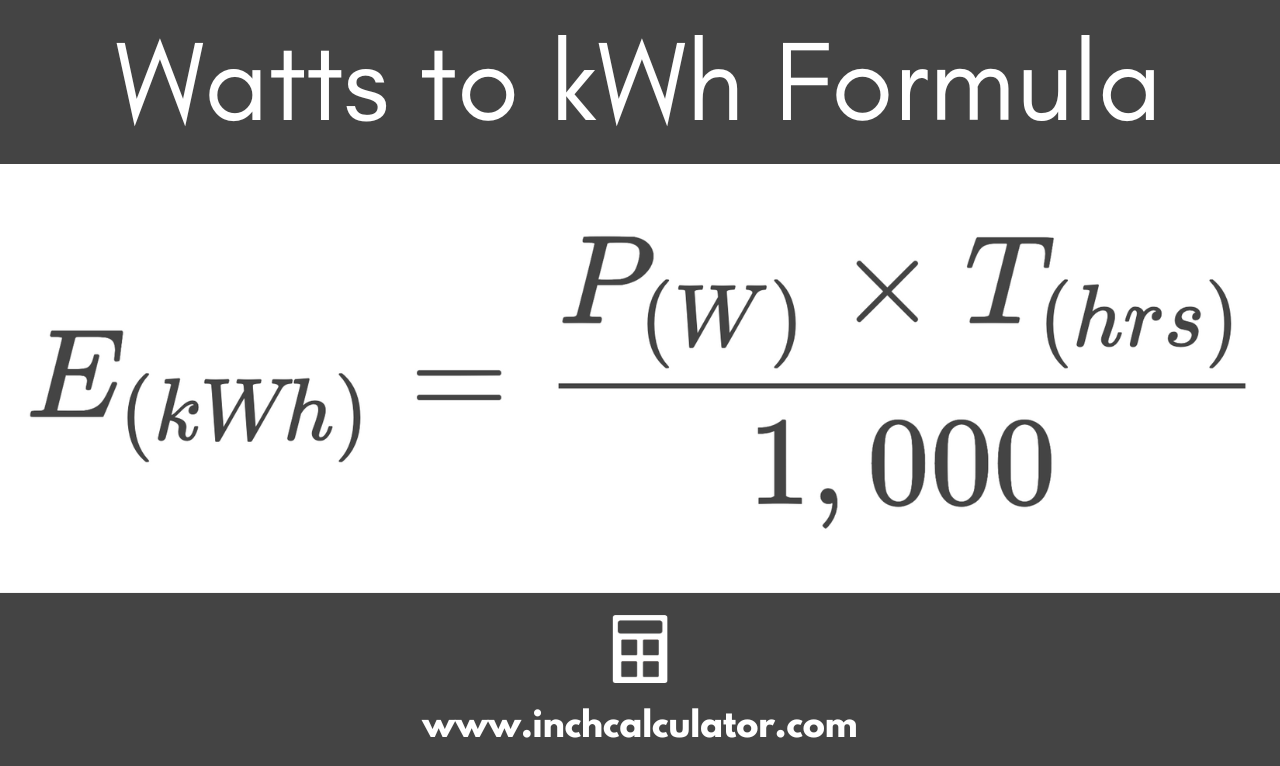# Watts to Kilowatt-Hours (kWh) Conversion Calculator

Convert watts to kilowatt-hours by entering power in watts and time in hours.

## Energy in Kilowatt-Hours:

kWh
Learn how we calculated this below

## How to Convert Watts to Kilowatt-Hours

A kilowatt-hour, expressed as kWh or kW·h, is a measure of energy that is equivalent to 1,000 watts of power for a 1-hour time period. Thus, to convert watts to kilowatt-hours, multiply the power in watts by the number of hours, then divide by 1,000.

### Watts to kWh Formula

Use the following formula to calculate energy in kilowatt-hours:

E(kWh) = P(W) × T(hrs) / 1,000

The energy E in kilowatt-hours is equal to the power P consumed in watts times the time T in hours, divided by 1,000.

For example: let’s find the kWh of 1,500 watts for 2.5 hours.

E(kWh) = 1,500 × 2.5 / 1,000
E(kWh) = 3,750 Wh / 1,000
E(kWh) = 3.75 kWh

Thus, the energy in kilowatt hours for a 1,500 power consumption over 2.5 hours is equal to 3.75 kilowatt-hours.

You can also use this formula to convert kilowatts to kilowatt-hours by not dividing by 1,000.Electricity usage is often measured in watt-hours or kilowatt-hours, so if you can convert watts to kWh, then you can predict how much an electric appliance might cost, or how much energy is required to power it.

For example: let’s predict how much it costs to power a light bulb every hour. A 100-watt light bulb uses 100 watts of power. To convert the power in watts to kilowatt-hours, multiply 100 watts by 1 hour, then divide by 1,000 to find the energy usage in kWh.

E(kWh) = 100 W × 1 hour / 1,000
E(kWh) = 100 Wh / 1,000
E(kWh) = 0.1 kWh

If electricity costs \$0.12 per kWh, then a 100-watt light bulb will cost 1.2 cents per hour that it’s on.

Try our electricity cost calculator to estimate the cost of power usage on your monthly bill.

## Common Watts to Kilowatt-Hour Conversions

Common watts to kilowatt-hour conversions for a 1-hour time period, along with the estimated cost of electricity, assuming a price of \$0.12 per kWh.
Power in Watts Energy in Kilowatt-hours Electricity Cost (at \$0.12/kWh)
100 W 0.1 kWh \$0.012 per hr
200 W 0.2 kWh \$0.024 per hr
300 W 0.3 kWh \$0.036 per hr
400 W 0.4 kWh \$0.048 per hr
500 W 0.5 kWh \$0.060 per hr
600 W 0.6 kWh \$0.072 per hr
700 W 0.7 kWh \$0.084 per hr
800 W 0.8 kWh \$0.096 per hr
900 W 0.9 kWh \$0.108 per hr
1000 W 1 kWh \$0.112 per hr

To convert energy to watts, use our kWh to watts conversion calculator.